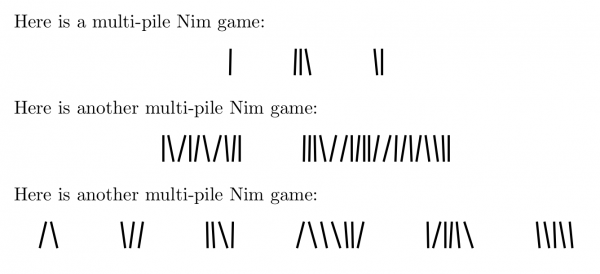# LaTeX for typesetting a multi-pile Nim game

I am preparing to teach our new final year module ‘Game Theory and Recreational Mathematics’. So I’m thinking about game typesetting in LaTeX (texlive-games is useful in this regard). I was looking for an easy way to display multi-pile Nim games. Usually, I find searching “latex thing” finds numerous options for typesetting “thing” in LaTeX, but here I was struggling.

Nim objects could be anything, of course, but conventionally sticks or stones are used. There are various types of dot in LaTeX that might look like stones, but somehow a line of dots didn’t seem satisfactory. There are various ways to draw a line (not least simply typing ‘|’), including some tally markers (e.g. in hhcount). My problem with these (call me picky) is that they are all identical lines, and a ‘heap’ of them just looks very organised. Really, I want a set of lines that looks like someone just threw them into heaps (though probably without crossings for the avoidance of ambiguity). So I wrote my own.

I used PGF/TikZ. My basic strategy was to draw a thick vertical line in TikZ with a little wobble added so each one doesn’t look extremely well-lined-up with its neighbour, achieved by adding or subtracting a small random number in PGF. This is a command \drawnimstick. On top of this, I made a command \nimgame{} which takes a comma-separated list of numbers and makes a row of Nim heaps with those numbers of sticks. For example, \nimgame{5,2,3} makes a 3-pile Nim game with five sticks in the first pile, two in the second and three in the third. There is no limit to the number of piles or the number in a pile, but this code doesn’t do anything to cope when line breaks start happening because I only anticipate using small numbers of small piles myself.

Here are the definitions of the commands \drawnimstick and \nimgame{}.

% load packages and seed random number
\usepackage{pgf,tikz}
\pgfmathsetseed{\number\pdfrandomseed}

% command \drawnimstick draws a single Nim stick with a little random wobble.
\newcommand{\drawnimstick}{%
\pgfmathsetmacro{\topx}{(random(0,20)-10)/100}% top of the line: 0 +/- random
\pgfmathsetmacro{\botx}{(random(0,20)-10)/100}% bottom of the line: 0 +/- random
\begin{tikzpicture}%
\draw[very thick] (\topx,0) -- (\botx,0.5);% draws line
\end{tikzpicture}%
}

% command \nimgame takes a comma-separated list of numbers and makes Nim heaps holding those number of sticks
\newcommand{\nimgame}{%
\begin{center}%
\def\listofgames{#1}%
\foreach \heap in \listofgames {% picks the numbers from the list
\foreach \index in {1, ..., \heap} {% loops for this heap
\drawnimstick\hspace{0.5mm}% draws a stick + 0.5mm space
}%
\hspace{10mm}% 10mm horizontal space between heaps
}%
\end{center}%
}


And here are some examples of it in action:

\documentclass{article}
...(the above definitions here)...
\begin{document}
% examples
Here is a multi-pile Nim game:
\nimgame{1,3,2}

Here is another multi-pile Nim game:
\nimgame{10,20}

Here is another multi-pile Nim game:
\nimgame{2,3,4,7,6,5}
\end{document}


Here is the output of the code above (of course, if you run it again, all the lines shift because the random parameters vary on run time):Tags: , ,

•